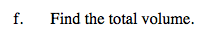### Home > CALC > Chapter 8 > Lesson 8.1.2 > Problem8-24

8-24.
1.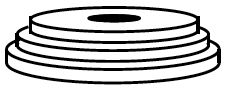While working out, Warren wanted to know the volume of the ten weights he was lifting. Each weight is a circular disk roughly 2 inches thick. Also, there is a circular hole (diameter 1 inch) at the center of each weight for the barbell. If the weights were stacked from largest to smallest, the radius of each disk isinch smaller than the next larger size. (Three of the weights are shown at right.) The largest weight has a diameter of 20 inches. The next weight has a diameter 19.5 inches, etc. Homework Help ✎

1. What is the radius of the largest disk?

2. What is the radius of the hole in the middle?

3. What is the formula for the volume of the largest disk, in words?

4. Write an expression for the volume of each disk if i represents the number of times the radius is reduced?

5. Use summation notation to write an expression that will find the volume of the weights he was lifting.

6. Find the total volume.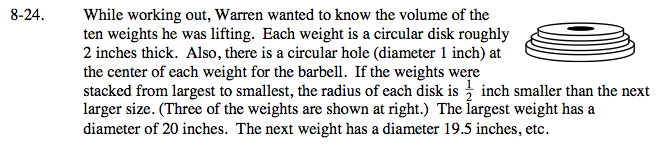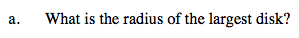The given information involved diameters, not radii.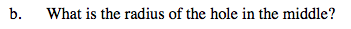Refer to hint in part (a).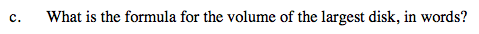Each disk is a cylinder with a hole in the middle. How would you compute the volume of such a figure?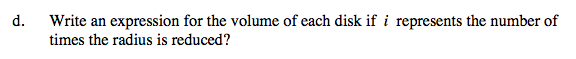$V=\pi \left ( 10-\frac{i}{2} \right )^{2}(2)-\pi \left ( \frac{1}{2} \right )^{2}(2)$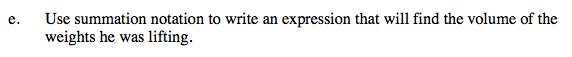Recall that the index will account for each and every weight. How many weights are there?